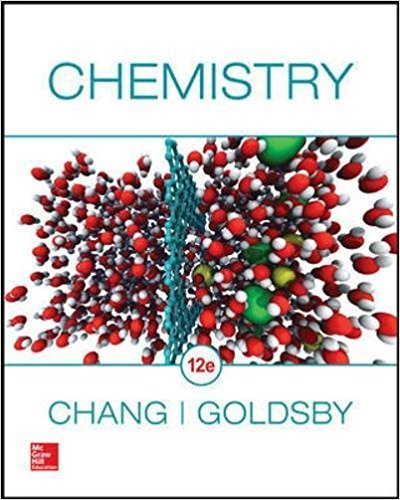×
Get Full Access to Chemistry - 12 Edition - Chapter 2 - Problem 2.4
Get Full Access to Chemistry - 12 Edition - Chapter 2 - Problem 2.4

×

# What is meant by the term fundamental particle?ISBN: 9780078021510 98

## Solution for problem 2.4 Chapter 2

Chemistry | 12th Edition

• Textbook Solutions
• 2901 Step-by-step solutions solved by professors and subject experts
• Get 24/7 help from StudySoup virtual teaching assistantsChemistry | 12th Edition

4 5 1 374 Reviews
25
1
Problem 2.4

What is meant by the term fundamental particle?

Step-by-Step Solution:

Step 1 of 2

A fundamental particle is a particle with no internal substance.

Step 2 of 2

##### ISBN: 9780078021510

Chemistry was written by and is associated to the ISBN: 9780078021510. The answer to “What is meant by the term fundamental particle?” is broken down into a number of easy to follow steps, and 8 words. Since the solution to 2.4 from 2 chapter was answered, more than 394 students have viewed the full step-by-step answer. This textbook survival guide was created for the textbook: Chemistry, edition: 12. The full step-by-step solution to problem: 2.4 from chapter: 2 was answered by , our top Chemistry solution expert on 09/09/17, 04:35AM. This full solution covers the following key subjects: . This expansive textbook survival guide covers 25 chapters, and 3241 solutions.

## Discover and learn what students are asking

Calculus: Early Transcendental Functions : Inverse Trigonometric Functions: Integration
?In Exercises 1-20, find the indefinite integral. $$\int \frac{1}{4+(x-3)^{2}} d x$$

Statistics: Informed Decisions Using Data : Estimating a Population Mean
?Explain what is meant by degrees of freedom.

Statistics: Informed Decisions Using Data : Inference about the Difference between Two Medians: Dependent Samples
?In Problems 3–10, use the Wilcoxon matched-pairs signedranks test to test the given hypotheses at the a = 0.05 level of significance. The dependent sa

Chemistry: The Central Science : Chemistry of the Nonmetals
What does hydrogen have in common with the halogens? Explain.

Unlock Textbook Solution

Enter your email below to unlock your verified solution to:

What is meant by the term fundamental particle?